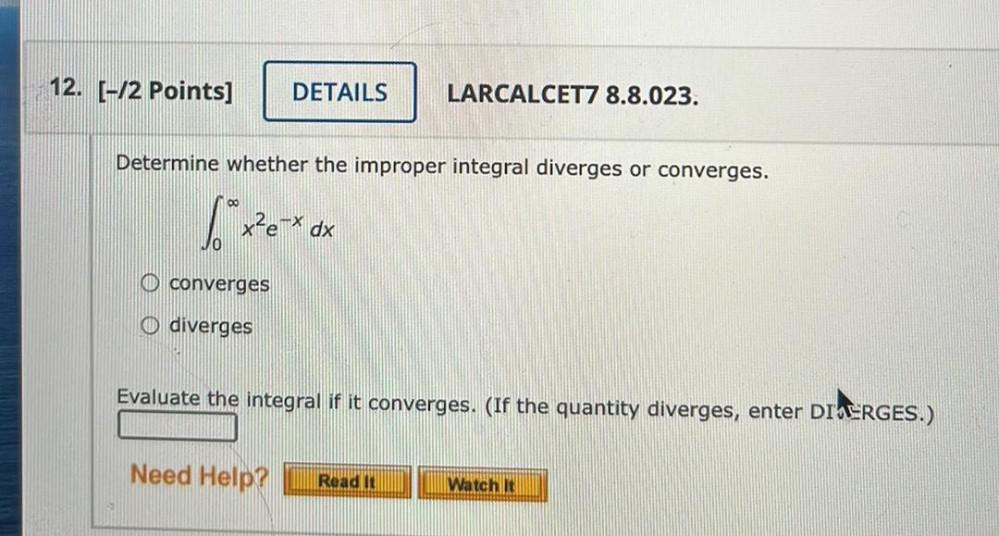Question:

# Determine whether the improper integral diverges or converges. ∞ ∫ x^2(e^-x)dx 0 a) converges b) diverges Evaluate the integral if it converges.Determine whether the improper integral diverges or converges. ∞ ∫ x^2(e^-x)dx 0 a) converges b) diverges Evaluate the integral if it converges. (If the quantity diverges, enter DIVERGES.)